• ## matlab imfill孔洞填充

万次阅读 2018-08-14 20:19:45
作用填充二值图像BW中的空洞   clear all; clc; close all; img = imread('test1.png'); if ndims(img)==3  img = rgb2gray(img); end img_bw = im2bw(img); img_fill = imfill(img_bw, 'holes'); ...
BW2 = imfill(BW)

作用填充二值图像BW中的空洞

clear all; clc; close all;

if ndims(img)==3

img = rgb2gray(img);

end

img_bw = im2bw(img);

img_fill = imfill(img_bw, 'holes');

figure;

subplot(1,2,1),imshow(img_bw), title('有空洞的图像');

subplot(1,2,2),imshow(img_fill), title('孔洞被填充的图像');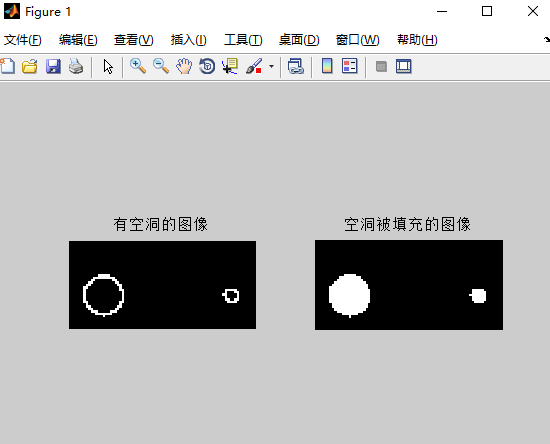展开全文• %先进行孔洞操作，再进行膨胀操作 %对复杂的图片效果好，而且要调试IBW=BW;或者IBW=~BW;看哪个更适合 I = imread('picture16.jpg'); BW=im2bw(I,0.4); IBW=~BW; F1 = imfill(IBW,'holes');SE = ones(3);F2 = ...


%先用canny，然后进行孔洞操作，再进行膨胀操作
%对简单的图片效果好
I=imresize(I,[600,600]);
I=rgb2gray(I);
BW = edge(I,'canny') ;
IBW=BW;
F1 = imfill(IBW,'holes');SE = ones(3);F2 = imdilate(F1,SE,'same');BW3 = bwperim(F2);
BW4=imfill(BW3,'holes');
figure,imshow(I);
figure,imshow(BW3);
figure,imshow(BW4);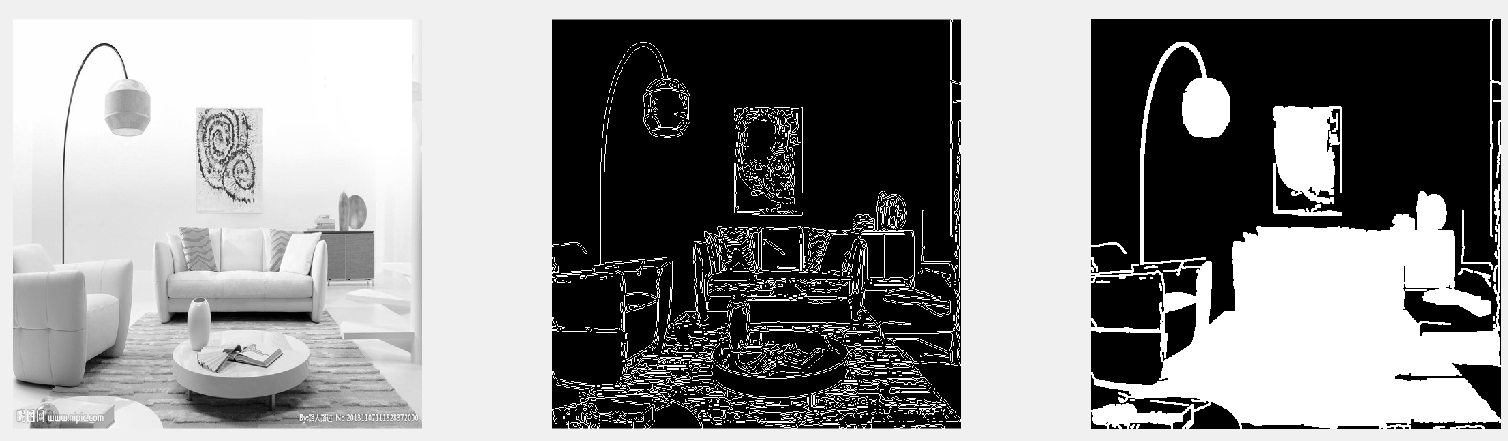%先进行孔洞操作，再进行膨胀操作
%对复杂的图片效果好，而且要调试IBW=BW;或者IBW=~BW;看哪个更适合
BW=im2bw(I,0.4);
IBW=~BW;
F1 = imfill(IBW,'holes');SE = ones(3);F2 = imdilate(F1,SE,'same');BW3 = bwperim(F2);
BW4=imfill(BW3,'holes');
figure,
subplot(1,3,1),imshow(I);
subplot(1,3,2),imshow(BW3);
subplot(1,3,3),imshow(BW4);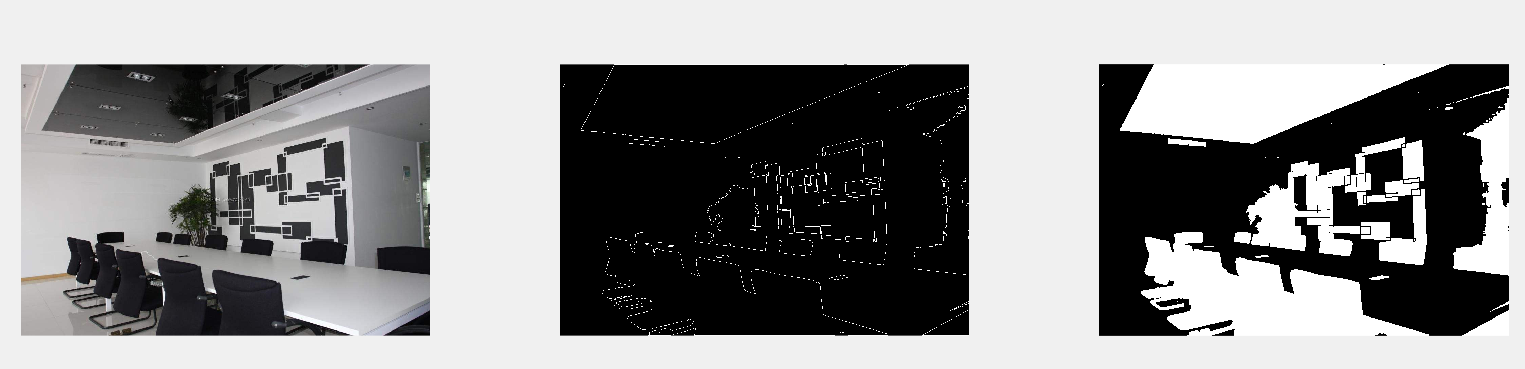展开全文• 在平时处理二值图像的时候，除了要进行形态学的一些操作，还有有上一节讲到的轮廓连通区域的面积周长标记等，还有一个最常见的就是孔洞填充，opencv这里成为漫水填充，其实也可以叫种子填充，或者区域生长，基本的...
可以说从这篇文章开始，就结束了图像识别的入门基础，来到了第二阶段的学习。在平时处理二值图像的时候，除了要进行形态学的一些操作，还有有上一节讲到的轮廓连通区域的面积周长标记等，还有一个最常见的就是孔洞的填充，opencv这里成为漫水填充，其实也可以叫种子填充，或者区域生长，基本的原理是一样的，但是应用的时候需要注意一下，种子填充用递归的办法，回溯算法，漫水填充使用堆栈，提高效率，同时还提供了一种方式是扫描行。经常用来填充孔洞，现在来具体看看。漫水填充：也就是用一定颜色填充联通区域，通过设置可连通像素的上下限以及连通方式来达到不同的填充效果;漫水填充经常被用来标记或分离图像的一部分以便对其进行进一步处理或分析，也可以用来从输入图像获取掩码区域，掩码会加速处理过程，或只处理掩码指定的像素点，操作的结果总是某个连续的区域。简单的说，就是选中点seedPoint，然后选取出它周围和它色彩差异不大的点，并将它们的值改为newVal。如果被选取的点，遇到mask掩码，则放弃对该方向的种子填充算法，种子填充算法是从多边形区域内部的一点开始，由此出发找到区域内的所有像素。种子填充算法采用的边界定义是区域边界上所有像素具有某个特定的颜色值，区域内部所有像素均不取这一特定颜色，而边界外的像素则可具有与边界相同的颜色值。具体算法步骤：标记种子(x,y)的像素点 ;检测该点的颜色，若他与边界色和填充色均不同，就用填充色填   充该点，否则不填充 ;检测相邻位置，继续 2。这个过程延续到已经检测区域边界范围内的所有像素为止。当然在搜索的时候有两种检测相邻像素：四向连通和八向连通。四向连通即从区域上一点出发，通过四个方向上、下、左、右来检索。而八向连通加上了左上、左下、右上、右下四个方向。这种算法的有点是算法简单，易于实现，也可以填充带有内孔的平面区域。但是此算法需要更大的存储空间以实现栈结构，同一个像素多次入栈和出栈，效率低，运算量大。扫描线种子填充算法:该算法属于种子填充算法，它是以扫描线上的区段为单位操作。所谓区段，就是一条扫描线上相连着的若干内部象素的集合。扫描线种子填充算法思想：首先填充当前扫描线上的位于给定区域的一区段，然后确定于这一区段相邻的上下两条线上位于该区域内是否存在需要填充的新区段，如果存在，则依次把他们保存起来，反复这个过程，直到所保存的各区段都填充完毕。FloodFill函数C++: int floodFill(InputOutputArray image, InputOutputArray mask,Point seedPoint, Scalar newVal, Rect* rect=0, Scalar loDiff=Scalar(), Scalar upDiff=Scalar(), int flags=4 );InputOutputArray:输入和输出图像。mask:            输入的掩码图像。seedPoint：      算法开始处理的开始位置。newVal：         图像中所有被算法选中的点，都用这个数值来填充。rect:            最小包围矩阵。loDiff：         最大的低亮度之间的差异。upDiff：         最大的高亮度之间的差异。flag：           选择算法连接方式。根据上面的函数先看一个基础的应用#include "opencv2/imgproc/imgproc.hpp"#include "opencv2/highgui/highgui.hpp"#include using namespace cv;using namespace std;Mat g_srcImage, g_dstImage, g_grayImage, g_maskImage;int g_nFillMode = 1;int g_nLowDifference = 20, g_nUpDifference = 20;int g_nConnectivity = 4;int g_bIsColor = true;bool g_bUseMask = false;int g_nNewMaskVal = 255;static void onMouse( int event, int x, int y, int, void* ){if( event != EVENT_LBUTTONDOWN )return;Point seed = Point(x,y);int LowDifference = g_nFillMode == 0 ? 0 : g_nLowDifference;int UpDifference = g_nFillMode == 0 ? 0 : g_nUpDifference;int flags = g_nConnectivity + (g_nNewMaskVal << 8) +(g_nFillMode == 1 ? FLOODFILL_FIXED_RANGE : 0);int b = (unsigned)theRNG() & 255;int g = (unsigned)theRNG() & 255;int r = (unsigned)theRNG() & 255;Rect ccomp;Scalar newVal = g_bIsColor ? Scalar(b, g, r) : Scalar(r*0.299 + g*0.587 + b*0.114);Mat dst = g_bIsColor ? g_dstImage : g_grayImage;//目标图的赋值int area;if( g_bUseMask ){threshold(g_maskImage, g_maskImage, 1, 128, THRESH_BINARY);area = floodFill(dst, g_maskImage, seed, newVal, &ccomp, Scalar(LowDifference, LowDifference, LowDifference),Scalar(UpDifference, UpDifference, UpDifference), flags);imshow( "mask", g_maskImage );}else{area = floodFill(dst, seed, newVal, &ccomp, Scalar(LowDifference, LowDifference, LowDifference),Scalar(UpDifference, UpDifference, UpDifference), flags);}imshow("效果图", dst);cout << area << " 个像素被重绘\n";}int main( int argc, char** argv ){g_srcImage = imread("lena.jpg", 1);if( !g_srcImage.data ) { printf("读取图片image0错误~！ \n"); return false; }g_srcImage.copyTo(g_dstImage);cvtColor(g_srcImage, g_grayImage, COLOR_BGR2GRAY);g_maskImage.create(g_srcImage.rows+2, g_srcImage.cols+2, CV_8UC1);namedWindow( "效果图",WINDOW_AUTOSIZE );createTrackbar( "负差最大值", "效果图", &g_nLowDifference, 255, 0 );createTrackbar( "正差最大值" ,"效果图", &g_nUpDifference, 255, 0 );setMouseCallback( "效果图", onMouse, 0 );while(1){//先显示效果图imshow("效果图", g_bIsColor ? g_dstImage : g_grayImage);//获取键盘按键int c = waitKey(0);//判断ESC是否按下，若按下便退出if( (c & 255) == 27 ){cout << "程序退出...........\n";break;}//根据按键的不同，进行各种操作switch( (char)c ){//如果键盘“1”被按下，效果图在在灰度图，彩色图之间互换case '1':if( g_bIsColor )//若原来为彩色，转为灰度图，并且将掩膜mask所有元素设置为0{cout << "键盘“1”被按下，切换彩色/灰度模式，当前操作为将【彩色模式】切换为【灰度模式】\n";cvtColor(g_srcImage, g_grayImage, COLOR_BGR2GRAY);g_maskImage = Scalar::all(0);//将mask所有元素设置为0g_bIsColor = false;//将标识符置为false，表示当前图像不为彩色，而是灰度}else//若原来为灰度图，便将原来的彩图image0再次拷贝给image，并且将掩膜mask所有元素设置为0{cout << "键盘“1”被按下，切换彩色/灰度模式，当前操作为将【彩色模式】切换为【灰度模式】\n";g_srcImage.copyTo(g_dstImage);g_maskImage = Scalar::all(0);g_bIsColor = true;//将标识符置为true，表示当前图像模式为彩色}break;//如果键盘按键“2”被按下，显示/隐藏掩膜窗口case '2':if( g_bUseMask ){destroyWindow( "mask" );g_bUseMask = false;}else{namedWindow( "mask", 0 );g_maskImage = Scalar::all(0);imshow("mask", g_maskImage);g_bUseMask = true;}break;//如果键盘按键“3”被按下，恢复原始图像case '3':cout << "按键“3”被按下，恢复原始图像\n";g_srcImage.copyTo(g_dstImage);cvtColor(g_dstImage, g_grayImage, COLOR_BGR2GRAY);g_maskImage = Scalar::all(0);break;//如果键盘按键“4”被按下，使用空范围的漫水填充case '4':cout << "按键“4”被按下，使用空范围的漫水填充\n";g_nFillMode = 0;break;//如果键盘按键“5”被按下，使用渐变、固定范围的漫水填充case '5':cout << "按键“5”被按下，使用渐变、固定范围的漫水填充\n";g_nFillMode = 1;break;//如果键盘按键“6”被按下，使用渐变、浮动范围的漫水填充case '6':cout << "按键“6”被按下，使用渐变、浮动范围的漫水填充\n";g_nFillMode = 2;break;//如果键盘按键“7”被按下，操作标志符的低八位使用4位的连接模式case '7':cout << "按键“7”被按下，操作标志符的低八位使用4位的连接模式\n";g_nConnectivity = 4;break;//如果键盘按键“8”被按下，操作标志符的低八位使用8位的连接模式case '8':cout << "按键“8”被按下，操作标志符的低八位使用8位的连接模式\n";g_nConnectivity = 8;break;}}return 0;}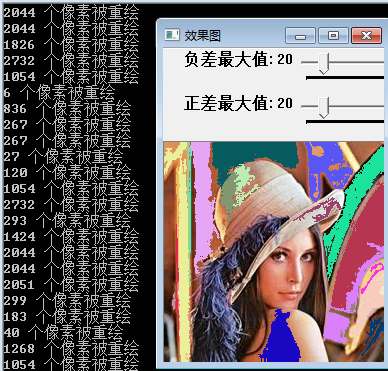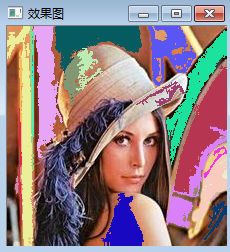再来看看漫水填充在填充孔洞上的应用#include#include#includeusing namespace std;using namespace cv;void chao_fillHole(const cv::Mat srcimage, cv::Mat &dstimage){Size m_Size = srcimage.size();Mat temimage = Mat::zeros(m_Size.height + 2, m_Size.width + 2, srcimage.type());srcimage.copyTo(temimage(Range(1, m_Size.height + 1), Range(1, m_Size.width + 1)));floodFill(temimage, Point(0, 0), Scalar(255));Mat cutImg;temimage(Range(1, m_Size.height + 1), Range(1, m_Size.width + 1)).copyTo(cutImg);dstimage = srcimage | (~cutImg);}int main(){Mat src=imread("111.png");Mat dst;chao_fillHole(src, dst)  ;imshow("tianchong",dst);waitKey(0);return 0;}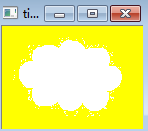matlabI=imread('tire.tif');figure,imshow(I)BW=imfill(I);figure,imshow(BW)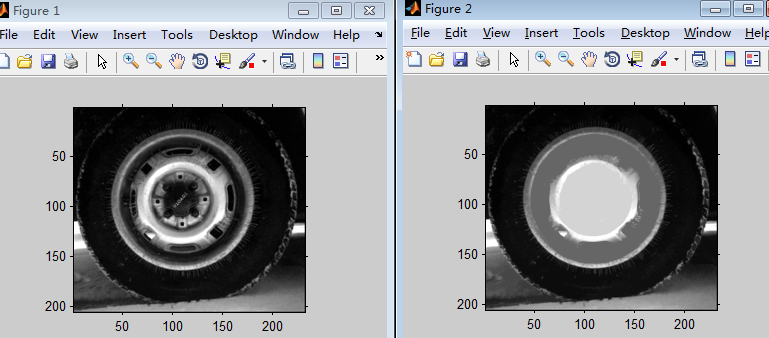图像识别算法交流 QQ群：145076161，欢迎图像识别与图像算法，共同学习与交流原文：http://blog.csdn.net/qq_20823641/article/details/52186629
展开全文• matlab中的imfill函数可以方便得实现二值图像的孔洞填充，而在opencv中并没有相同功能的函数。因此，在opencv的基础上编写实现孔洞填充的函数，并且能够设定阈值，对面积大于阈值的孔洞不进行填充。使用形态学重建的...
      matlab中的imfill函数可以方便得实现二值图像的孔洞填充，而在opencv中并没有相同功能的函数。因此，在opencv的基础上编写实现孔洞填充的函数，并且能够设定阈值，对面积大于阈值的孔洞不进行填充。使用形态学重建的算法能够有效地实现孔洞填充，具体算法参照《数字图像处理》第三版9.5.9节，孔洞填充。    主要实现代码如下所示：其中imfill函数即为空洞填充的实现函数，第一个参数是二值图像（0~1），第二个参数是填充孔洞的阈值。若孔洞面积大于阈值则不填充，反之则填充。#include "iostream"
#include <opencv2\opencv.hpp>
using namespace std;
using namespace cv;
Mat inv_board(Mat src);
Mat inv_img(Mat src);
void delarea(Mat& bw, int max);
Mat imfill(Mat I, int max);
void main()
{
Mat I, src_gray, F_B, F_BI_C, temp, H, I_fill;
cvtColor(scr, src_gray, COLOR_BGR2GRAY);
threshold(src_gray, I, 0.1, 1,0);
I_fill = imfill(I,40);
imshow("原二值图", I * 255);
imshow("填充图", I_fill*255);
waitKey(0);
}

Mat imfill(Mat I,int max)
{
Mat  src_gray, F_B, F_BI_C, temp, H, I_fill;
I_fill = I.clone();
Mat F = inv_board(I);
Mat I_C = inv_img(I);
Mat element = getStructuringElement(0, Size(3, 3), Point(1, 1));
while (1)
{

dilate(F, F_B, element);
F_BI_C = F_B.mul(I_C);
temp = F_BI_C - F;
if (sum(temp) == Scalar(0))
break;
else
F = F_BI_C.clone();
}
H = inv_img(F_BI_C);
Mat H_IC = H.mul(I_C);
delarea(H_IC, max);
for (int i = 0; i < H_IC.rows; i++)
{
for (int j = 0; j < H_IC.cols; j++)
{
if (H_IC.at<uchar>(i, j) == 1)
I_fill.at<uchar>(i, j) = 1;
}
}
return I_fill;
}
Mat inv_board(Mat src)
{

int rows = src.rows;
int cols = src.cols;
Mat dst = Mat::zeros(rows, cols, CV_8UC1);
for (int i = 0; i < cols; i++)
{
dst.at<uchar>(0, i) = 1 - src.at<uchar>(0, i);
}
for (int i = 0; i < cols; i++)
{
dst.at<uchar>(rows-1, i) = 1 - src.at<uchar>(rows - 1, i);
}
for (int i = 1; i < rows-1; i++)
{
dst.at<uchar>(i, 0) = 1 - src.at<uchar>(i, 0);
}
for (int i = 1; i < rows - 1; i++)
{
dst.at<uchar>(i, cols-1) = 1 - src.at<uchar>(i, cols-1);
}
return dst;
}

Mat inv_img(Mat src)
{
int rows = src.rows;
int cols = src.cols;
Mat dst = src.clone();
for (int i = 0; i < rows; i++)
for (int j = 0; j < cols; j++)
dst.at<uchar>(i, j) = 1 - src.at<uchar>(i, j);
return dst;
}

void delarea(Mat& bw, int max )
{
Mat bw_copy = bw.clone();
int flag = 0;
Mat H_b, H_bw, temp;
Mat H = Mat::zeros(bw.size(), bw.type());
for (int i = 0; i < bw.rows; i++)
{
for (int j = 0; j < bw.cols; j++)
{
if (bw_copy.at<uchar>(i, j) == 1)
{
H.at<uchar>(i, j) = 1;
Mat element = getStructuringElement(0, Size(3, 3), Point(1, 1));
while (1)
{
dilate(H, H_b, element);
H_bw = H_b.mul(bw);
temp = H_bw - H;
if (sum(temp) == Scalar(0))
break;
else
H = H_bw.clone();
}
bw_copy = bw_copy - H_bw;
if (sum(H_bw).val > max)
{
bw = bw - H_bw;
}
H = Mat::zeros(bw.size(), bw.type());
}

}
}
}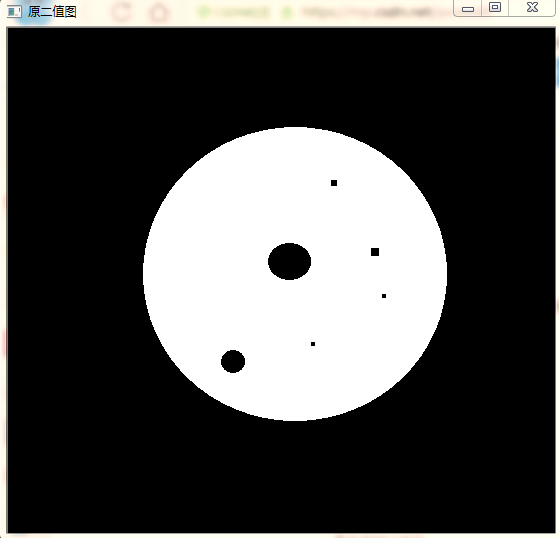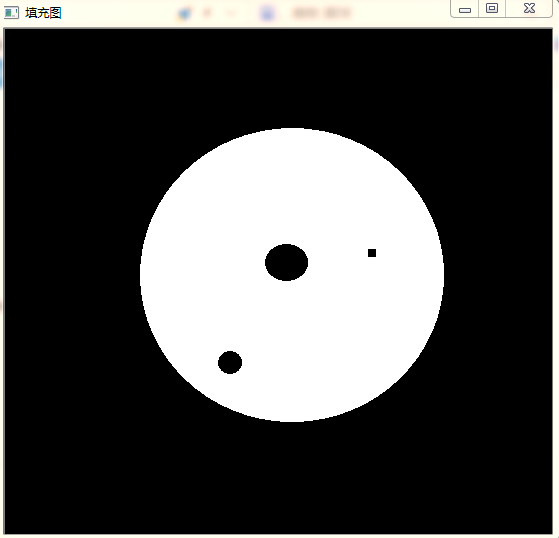展开全文图像处理
• Matlab下，使用imfill可以很容易的完成孔洞填充操作，感觉这是一个极为常用的方法，然而不知道为什么OpenCV里面却没有集成这个函数。在网上查了好多关于Opencv下的孔洞填充方法，大部分使用轮廓查找方法去做的，但...
• opencv 孔洞填充，方案1：  http://bbs.csdn.net/topics/391542633?page=1 opencv 孔洞填充，方案1：  其实主要是imfill(matrix, 'holes'); 的openCV/C++ 实现。 Overview: imfill...
• 使用opencv实现matlab中的imfill填充孔洞功能，整体思路如下： 1. 首先给原始图像四周加一圈全0，并保存为另一幅图像 2. 使用floodFill函数给新图像进行填充，种子点设置为Point(0, 0)，填充颜色为全白。因为...
• 使用opencv实现matlab中的imfill填充孔洞功能，整体思路如下：1.首先给原始图像四周加一圈全0，并保存为另一幅图像2.使用floodFill函数给新图像进行填充，种子点设置为Point(0, 0)，填充颜色为全白。因为原始图像...
• 填充二值图像的孔洞是一个非常常见的方法，因为某些原因我不得不用python来写我的程序，当我想要使用类似于MATLAB 中的imfill 方法时， 我最初发现的是opencv 中的cv2.floodFill 的方法，其实现的过程有点麻烦，而且...
• 1. 方法1： ... 然后用填充后的图减去原图，得到孔洞图； c. 最后用bwperim(Enclosed_bw_Img,8)函数提取孔洞边界轮廓。 2. 方法2： 采用Flood-Fill 泛洪算法。具体需要查找资料深入了解。 ...计算机视觉 图像处理
• ## OpenCV空洞填充算法

万次阅读 2015-08-16 11:40:20
Matlab下，使用imfill可以很容易的完成孔洞填充操作，感觉这是一个极为常用的方法，然而不知道为什么Opencv里面却没有集成这个函数。在网上查了好多关于Opencv下的孔洞填充方法，大部分使用轮廓查找方法去做的，但...OpenCV
• Matlab下，使用imfill可以很容易的完成孔洞填充操作，感觉这是一个极为常用的方法，然而不知道为什么Opencv里面却没有集成这个函数。在网上查了好多关于Opencv下的孔洞填充方法，大部分使用轮廓查找方法去做的，...
• 讨论帖：在Matlab下，使用imfill可以很容易的完成孔洞填充操作，感觉这是一个极为常用的方法，然而不知道为什么Opencv里面却没有集成这个函数。在网上查了好多关于Opencv下的孔洞填充方法，大部分使用轮廓查找方法去...
• 为底层纹理创建二值掩膜图像①分割后的图像目标区域显示为白色；②与原图像相比，图像顶部的纹理被过度分割，而底部纹理则以一...使用imfill函数对图像中的孔洞进行填充；%Step 3: Create Rough Mask for the Botto...
• 包括形态学运算、孔洞填充、平滑、标签等一系列操作，每个步骤均有注释，程序可读性强。 以下是代码片段： % imclose the image se=strel('disk',3); f6=imclose(f5,se); figure(4); imshow(f6); % fill the image ...
• ![图片说明]...!...!...我用imfill(bw,'holes');进行孔洞填充后无法去除与边界相连的伪目标，而且后面进行圆形度和面积计算时都会把图像边界给算进去，请问各位大神这个是怎么回事呀？求指导，本人将不胜感激呀！
• 孔洞填充 lbw=imfill(lbw); %填充孔洞 <p>%% 搜索甘蔗区域左侧边界，右侧边界 pos_l=zeros(1,2); pos_r=zeros(1,2); for i=1:m  tim=lbw(i,:); ...
• 在实际处理图像时，经常需要对...3、形态学处理：腐蚀、膨胀、孔洞填充。4、连通区域提取。主要涉及到的Matla图形处理函数如下：rgb2ycbcr（色彩空间转换），roicolor（ROI二值化），imerode（腐蚀），imdilate（膨...lable
• 慢慢更这篇，一边理解一边更，包括边界提取、孔洞填充(重点，因为我要考的课有道题就考这个)、骨架、连通分量、细化、裁剪等等。 1.边界提取   表示为 β(A)\beta(A)β(A) 的集合 AAA 的边界可以先用 BBB 对 AAA ...
• 为弥补二值化后图像中出现的断边、孔洞，借助Sobel算子进行边缘提取以接合断边，并基于数学形态学算子填充孔洞。再标签化处理图像并提取水果的颜色、形状、边缘特征，用200幅水果图像提取上述特征构造训练样本和测试...BP神经网络
• 　9.5.2 填充孔洞 　9.5.3 清除边界对象 　9.6 灰度图像形态学 　9.6.1 膨胀和腐蚀 　9.6.2 开运算和闭运算 　9.6.3 重构 　小结 第10章 图像分割 　前言 　10.1 点、线和边缘检测 　10.1.1 点检测 　10.1.2 线检测 ...
• 30使用OpenCV实现图像孔洞填充 - 31OpenCV_WarpTriangle 31使用OpenCV将一个三角形仿射变换到另一个三角形 - 32OpenCV_NonPhotorealisticRendering 32使用OpenCV进行非真实感渲染 - 33OpenCV_HoughTransform ...# matlab孔洞填充matlab 订阅